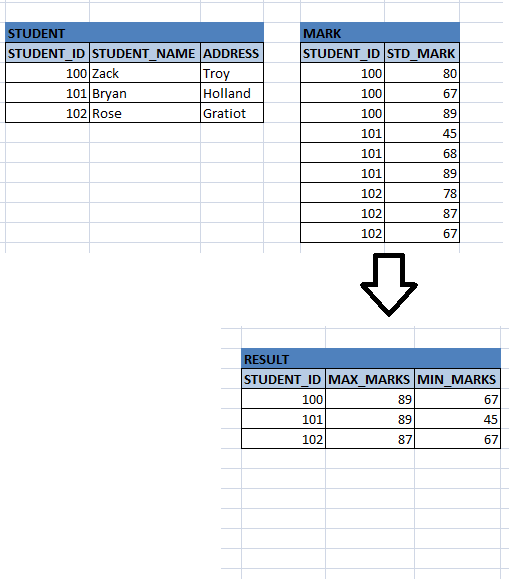Aggregation in DBMS

Suppose we want see the maximum and minimum marks of each student in class. What do we do in this case? We have to write an algorithm to fetch maximum and minimum marks of each student to be searched in class. But SQL provides a function to find this in the SELECT query itself. Hence it makes our task simpler.

In the SELECT query, we have to specify GROUP BY clause and mention the column based on which we need to find the maximum or minimum values. In the example above STUDENT_ID is the column to be specified after GROUP BY clause. We can even find the total number of records, sum of the numeric columns, and average of the column values based on the group of columns.

SELECT STUDENT _ID, MAX (std_mark) max_marks, MIN (std_mark) min_marks
FROM STUDENT s, MARKS m
WHERE s.STUENT_ID = m.STUDENT_ID
GROUP BY STUDENT _ID;Some of the Group functions are

• Count – it counts the total number of records in the table/s after applying ‘where’ clause. If where clause is not specified, it gives the total number of records in the table. If Group by clause is applied, it filters the records based on where clause (if any), then groups the records based on the columns in group by clause and gives the total count of records in each grouped category.

SELECT STUDENT _ID, SUM (std_mark) total_marks
FROM STUDENT s, MARKS m
WHERE s.STUENT_ID = m.STUDENT_ID
GROUP BY STUDENT _ID;

• AVG – It gives the average value of a column, provided column has numeric value. E.g.: Average age of students present in particular class.
File Processing System

SELECT STUDENT _ID, AVG (AGE) avg_age
FROM STUDENT s
WHERE s.CLASS_ID = ‘DESIGN_01’
GROUP BY STUDENT _ID;

SELECT STUDENT _ID, AVG (std_mark) avg_marks
FROM STUDENT s, MARKS m
WHERE s.STUENT_ID = m.STUDENT_ID
GROUP BY STUDENT _ID;

• MAX – It gives the maximum value in a column. For example, highest scorer in the class can be retrieved by MAX function.
• MIN– It gives the minimum value in a column. For example, lowest paid employee in a department can be obtained by MIN.

These group functions can be used with group by clause or without it

• Having Clause – Using this clause we can add conditions to the grouped categories and filter the records. For examples, if we want to display the details of the department which has more than 100 employees.

SELECT d.DEPATMENT_NAME, COUNT (e.DEPATMENT_ID) total_emp_count
FROM EMPLOYEE e, DEPARTMENT d
WHERE e.DEPARTMENT_ID = d.DEPARTMENT_ID – this condition pulls the matching employees
GROUP BY e.DEPARTMENT_ID
HAVING COUNT (e.DEPATMENT_ID)>100; -- filters more than 100 employees present in specific department.
We cannot give this condition in the where clause as this is the result of Group by clause.